Contents

# Shape sensitivity of energy

## Crack problems, J-integral, and GJ-integral

### 3-d fracture problems and GJ-integral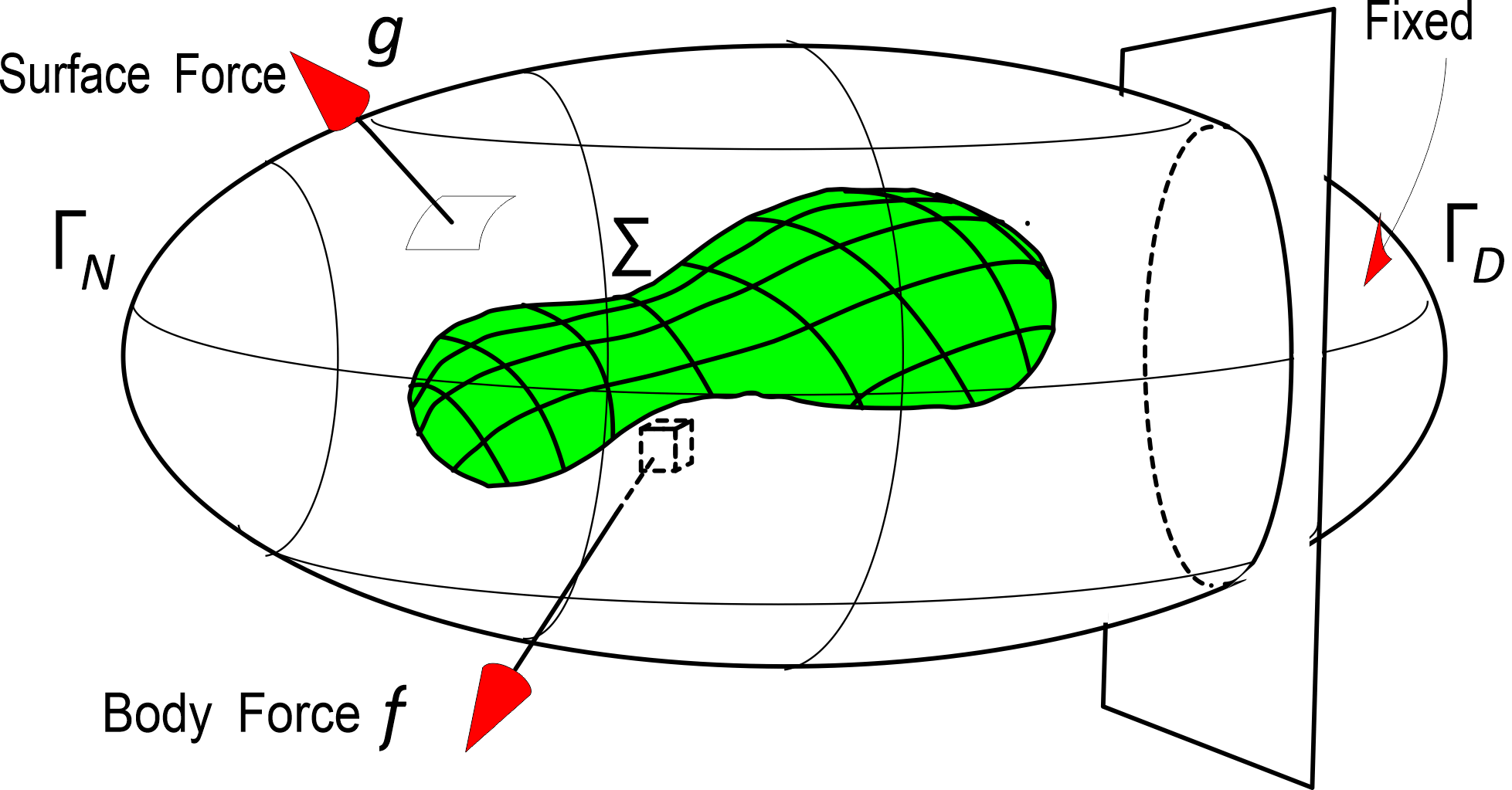Elastic body with the crack $\Sigma$
In the 3-dimensional case, $\Omega (t)=D\setminus \Sigma (t)$ with the domain $D$ in $\mathbb{R}^3$ with the Lipschitz property and the surface $\Sigma (t)\subset D$ with the edge $\partial \Sigma (t)$ (closed curve). Let us consider the minimizer $u(t)$ of (2.1) over $$V(\Omega (t))=\{v\in H^1(\Omega (t);\mathbb{R}^3):\, v=0 \textrm{on }\Gamma _D\}$$ where $\Gamma _D$ is the part of $\partial D$.
Smooth crack extension
Here we assumed that the crack extension is smooth as follows
SC1
There is a smooth 2-dimensional manifold $\Pi$ embedded in $\mathbb{R}^3$ such that $\Sigma (t)\subset \Pi , 0\le t\le T$.
SC2
$\Sigma =\Sigma (0)\subset \Sigma (t)\subset \Sigma (t')$ if $0 \lt t \lt t'$.
SC3
For each $t\in [0,T]$, there is a $C^{\infty }$-diffeomorphism $\phi _t:\partial \Sigma →\partial \Sigma (t)$ such that the map $[t\mapsto \phi _t]\in C^{\infty }([0,T];C^{\infty }(\partial \Sigma ;\Pi ))$.
SC4
The limit $\lim _{t→0}t^{-1}|\Sigma (t)-\Sigma |$ exists and nonzero.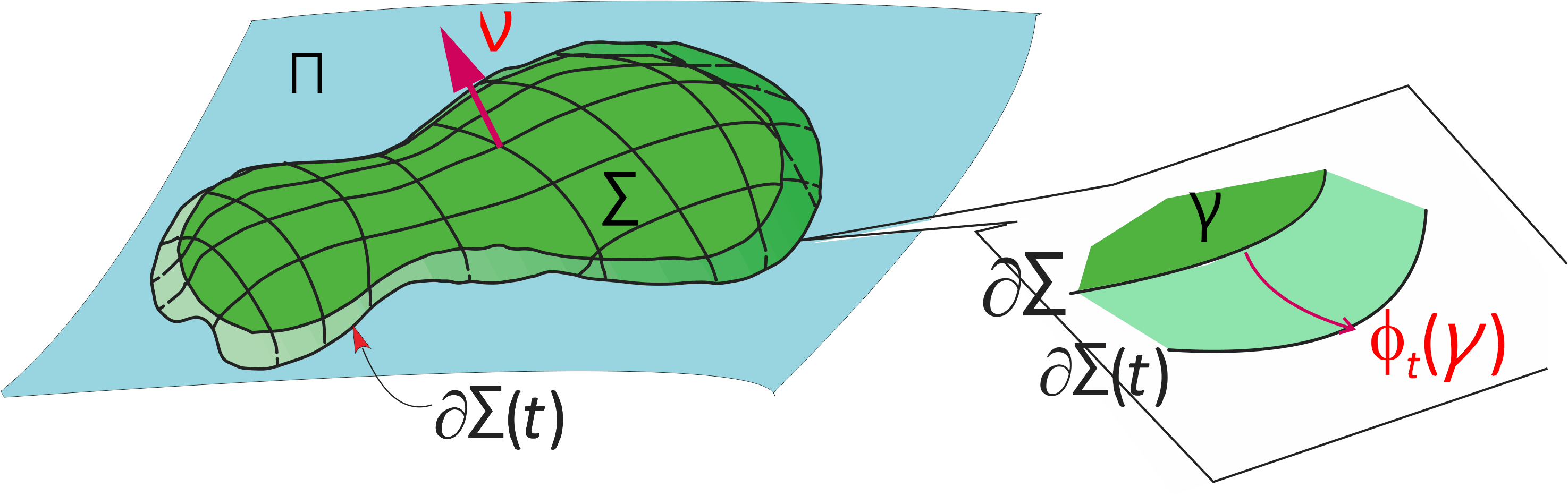Geodesic coordinate
We now consider the vector field $\mu _C(\gamma ), \gamma \in \partial \Sigma$ obtained from crack extension $[\gamma \mapsto \phi _t(\gamma )]$ and its extension $\mu _C(x)$ of $\mu _C(\gamma )$ defined in a neighborhood $x\in U(\partial \Sigma )$ of $\partial \Sigma$ using a geodesic coordinate perpendicular to $\partial \Sigma$ as follows.
1. Let $e_{\partial \Sigma ,1}(\gamma ), e_{\partial \Sigma ,2}(\gamma )$ be tangential vector fields at $\gamma \in \partial \Sigma$ on $\Pi$ such that $|e_{\partial \Sigma ,1}(\gamma )|=|e_{\partial \Sigma ,2}(\gamma )|=1$, $e_{\partial \Sigma ,2}(\gamma )$ tangent along the curve $\partial \Sigma$. Let us take $e_{\partial \Sigma ,1}(\gamma )$ in the crack extension direction and $e_{\partial \Sigma ,1}(\gamma )\perp e_{\partial \Sigma ,2}(\gamma )$.
2. There is a neighborhood $\tilde{U}(\Sigma )$ of $\Sigma$ such that there is only one nearest point ${\mathcal P}(x)\in \Pi$ for all $x\in \tilde{U}(\Sigma )$. Let us denote the distance from $x$ to ${\mathcal P}(x)\in \Pi$ by $\lambda _3(x)$, that is, $$x={\mathcal P}(x)+\lambda _3(x)e_{\Sigma ,3}({\mathcal P}(x)) e_{\Sigma ,3}(p)=-\nu (p),\, p\in \Pi$$ where $\nu (p)$ is the unit normal vector at $p\in \Pi$ in the direction from plus side to minus side of $\Pi$.
3. For each $\gamma \in \partial \Sigma$, consider the geodesic curve $[\lambda \mapsto g_{\Sigma }(\gamma ,\lambda )]$ satisfying the second order differential equation (the geodesic equation) with the initial conditions $$g_{\Sigma }(\gamma ,0)=\gamma , \frac{d g_{\Sigma }}{d\lambda }(\gamma ,0)=e_{\partial \Sigma ,1}(\gamma )$$ We can take a neighborhood $U(\partial \Sigma )$ of $\partial \Sigma , U(\partial \Sigma )\subset \tilde{U}(\Sigma )$ so that there is only one point $\gamma ({\mathcal P}(x))\in \partial \Sigma$ across $\partial \Sigma$ through ${\mathcal P}(x)$ by geodesic curve perpendicularly \cite [9.5]{Pr10};We now write the length of the geodesic curve from $\gamma ({\mathcal P(x)})$ to ${\mathcal P}(x)\in \Pi$ by $\lambda _1(x)$. \li There is a number $\epsilon \gt 0$ so that the mapping \begin{equation} F_{\partial \Sigma }:(\gamma ,\lambda _1,\lambda _3)\mapsto g_{\Sigma }(\gamma ,\lambda _1)+\lambda _3e_{\Sigma ,3}(g_{\Sigma }(\gamma ,\lambda _1)) \tag{2.21} \end{equation} become 1-1 mapping from $\partial \Sigma \times (-\epsilon ,\epsilon )^2$ into $\mathbb{R}^3$. Now we replace $U(\partial \Sigma )$ with $F_{\partial \Sigma }(\partial \Sigma \times (-\epsilon ,\epsilon )^2)$. Then $F_{\partial \Sigma }$ become the diffeomorphism from $\partial \Sigma \times (-\epsilon ,\epsilon )^2$ onto $U(\partial \Sigma )$.
4. Take $\omega$ so that $\overline{\omega }\subset U(\partial \Sigma )$.
5. We define the velocity of crack extension at the edge of crack by \begin{equation} v_{C}(\gamma ):=\left \langle \left .\frac{d}{dt}\phi _t(\gamma )\right |_{t=0},e_{\partial \Sigma ,1}(\gamma )\right \rangle ~~ \gamma \in \partial \Sigma \tag{2.22} \end{equation} where $\langle ,\rangle$ stands for the inner product in $\mathbb{R}^3$.
6. We now extend $v_C(\gamma )$ to the vector defined in $U(\partial \Sigma )$ by $$\mu _C(x):=v_{C}(\gamma ({\mathcal P}(x)))e_{\partial \Sigma ,1}(\gamma ({\mathcal P}(x))) x\in U(\partial \Sigma )$$ .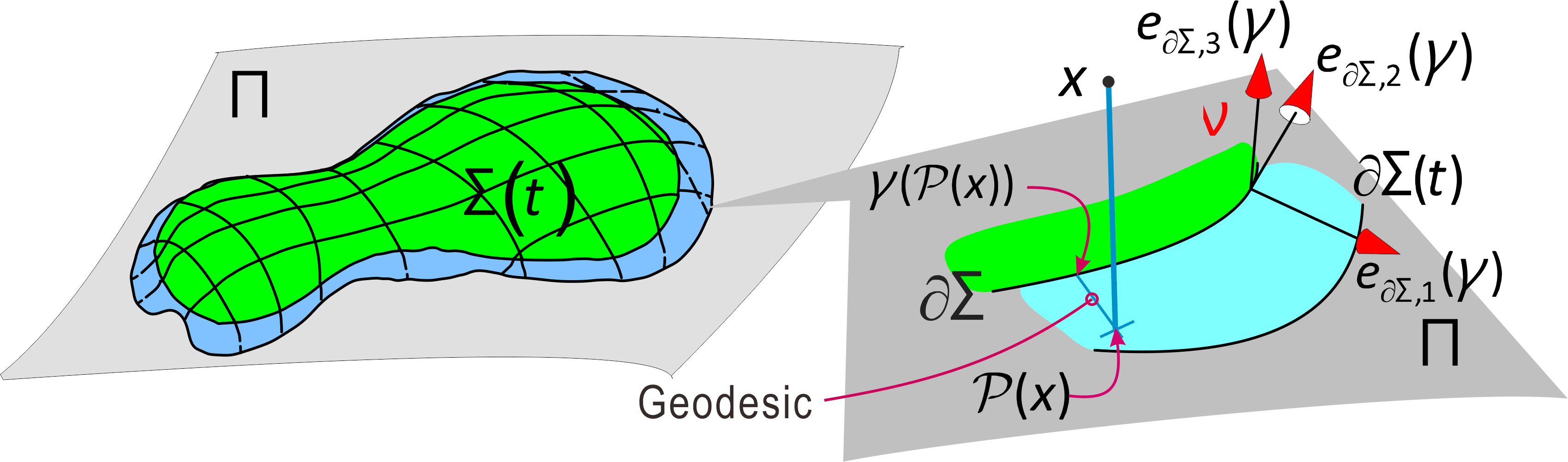Geodesic coordinate system near the crack front $\partial \Sigma$
We already have the following in [Theorem 5.5, Oh81].
Theorem 2.7
For a smooth crack extension $\{\Sigma (t)\}$, \begin{eqnarray} \mathcal{G}(f,g,\Sigma (\cdot )) &=&J_{\omega }(u,\mu _C |\partial \omega )\left (\int _{\partial \Sigma }v_{C}(\gamma )d\gamma \right )^{-1} \tag{2.23} \end{eqnarray} \begin{eqnarray} \mathcal{G}(f,g,\Sigma (\cdot ))&:=& \lim _{t→+0}\frac{\mathcal{E}(u;f,g,\Omega )-\mathcal{E}(u(t);f,g,\Omega (t))}{|\Sigma (t)\setminus \Sigma |_{\Pi }}\notag \tag{2.24} \end{eqnarray} where $|\cdot |_{\Pi }$ is the surface measure on $\Pi$ and $d\gamma$ the line element of $\partial \Sigma$.
For 3-dimentional fracture, see [Oh02-2] for more.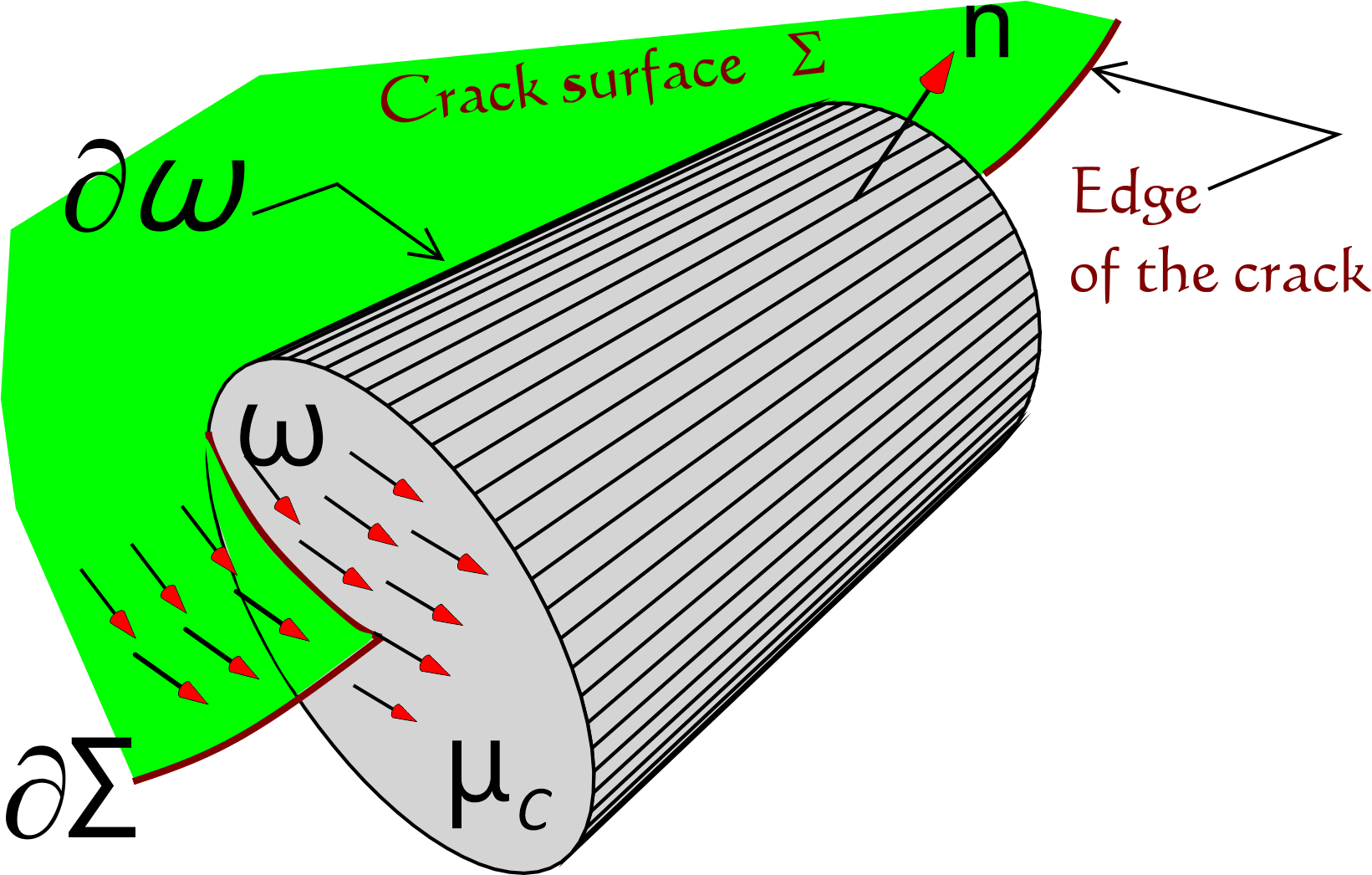A part of a tubular neighborhood $\omega$ of the crack front $\partial \Sigma$ and the vector field $\mu _C$ obtained from the crack extension

Information about the page: The current position is painted circle in the diagram below. Blue is the main MaKR and orange is a duplicate for MaKR's public use, where dashed line means the connection to the private area The dashed lines are only connections to main MaKR.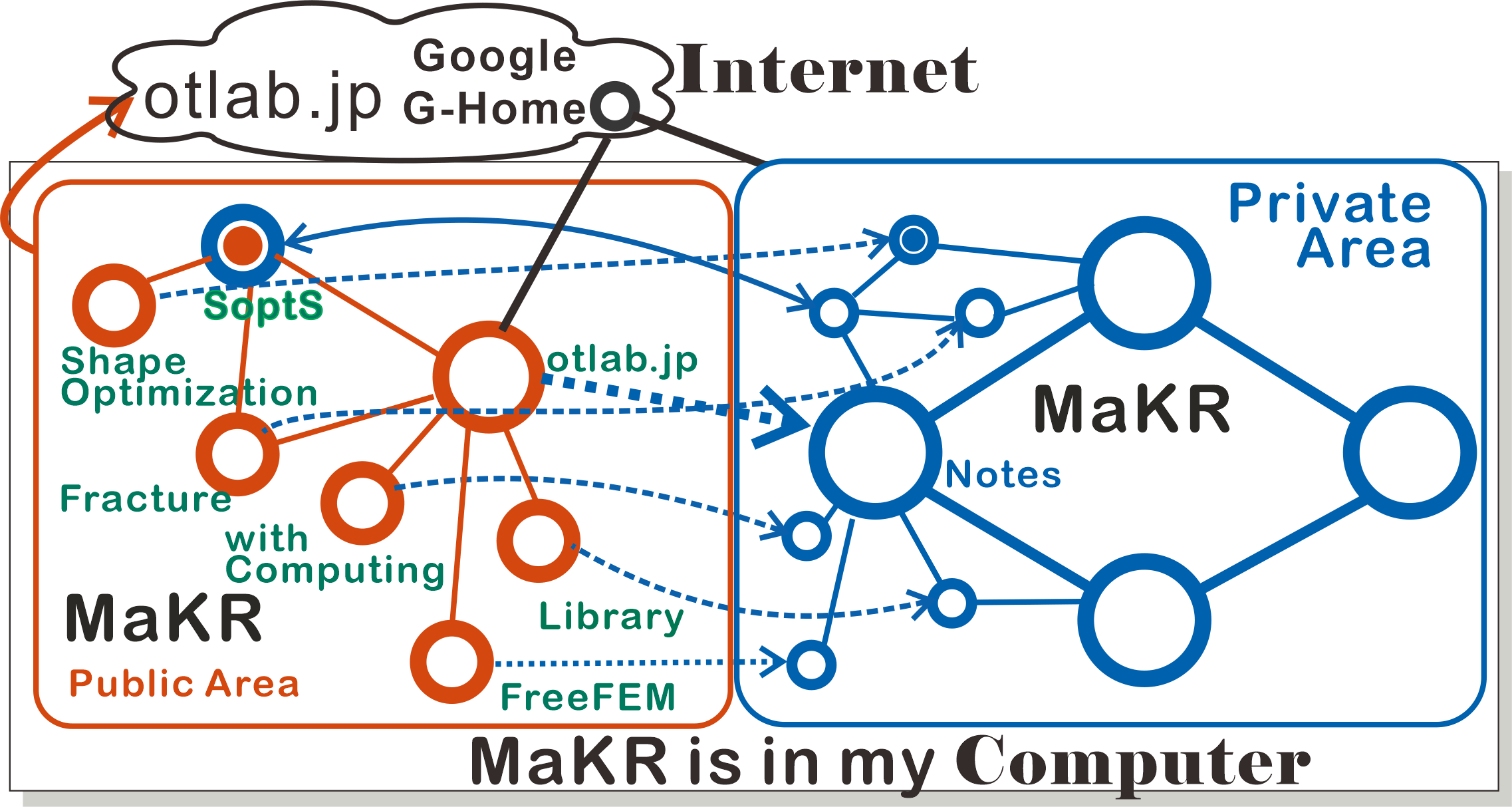[A-P06] G. Allaire and O. Pantz, Structural optimization with FreeFem++, Struct. Multidiscip. Opt, 32 (2006), 173--181.
[Al07] G. Allaire, Conception optimale de structures, Springer, 2007.
[Az94] H. Azegami, Solution to domain optimization problems, Trans. Japan Soc. Mech. Engrs. Series A, 60, No.574 (1994), 1479--1486. (in Japanese)
[A-W96] H. Azegami and Z. Wu, Domain optimization analysis in linear elastic problems: Approach using traction method, JSME Inter. J. Series A, 39 (1996), 272--278.
[Az17] H. Azegami. Solution of shape optimization problem and its application to product design, Mathematical Analysis of Continuum Mechanics and Industrial Applications, Springer, 2017, 83--98.
[B-S04] M.P. Bends{\o }e and O. Sigmund, Topology optimization: theory, methods, and applications, Springer, 2004.
[Bu04] H.D. Bui, Fracture mechanics -- Inverse problems and solutions, Springer, 2006.
[Ch67] G.P. Cherepanov, On crack propagation in continuous media, Prikl. Math. Mekh., 31 (1967), 476--493.
[Cir88] P.G. Ciarlet, Mathematical elasticity: Three-dimensional elasticity, North-Holland, 1988.
[Co85] R. Correa and A. Seeger, Directional derivative of a minimax function. Nonlinear Anal., 9(1985), 13--22.
[D-Z88] M.C. Delfour and J.-P. Zolésio, Shape sensitivity analysis via min max differentiability, SIAM J. Control and Optim., 26(1988), 834--862.
[D-D81] Ph. Destuynder and M. Djaoua, Sur une interprétation de l'intégrale de Rice en théorie de la rupture fragile. Math. Meth. in Appl. Sci., 3 (1981), 70--87.
[E-G04] A. Em and J.-L. Guermond, Theory and practice of finite elements, Springer, 2004.
[Es56] J.D. Eshelby, The Continuum theory of lattice defects, Solid State Physics, 3 (1956), 79--144.
[F-O78] D. Fujiwara and S. Ozawa, The Hadamard variational formula for the Green functions of some normal elliptic boundary value problems, Proc. Japan Acad., 54 (1978), 215--220.
[G-S52] P.R. Garabedian and M. Schiffer, Convexity of domain functionals, J.Anal.Math., 2 (1952), 281--368.
[Gr21] A.A. Griffith, The phenomena of rupture and flow in solids, Phil. Trans. Roy. Soc. London, Series A 221 (1921), 163--198.
[Gr24] A.A. Griffith, The theory of rupture, Proc. 1st.Intern. Congr. Appl. Mech., Delft (1924) 55--63.
[Gr85] P. Grisvard, Elliptic problems in nonsmooth domains, Pitman, 1985.
[Gr92] P. Grisvard, Singularities in boundary value problems, Springer, 1992.
[Had68] J. Hadamard, Mémoire sur un problème d'analyse relatif à l'équilibre des plaques élastiques encastrées, Mémoire des savants étragers, 33 (1907), 515--629.
[Hau86] E.J. Haug, K.K. Choi and V. Komkov, Design sensitivity analysis of structural systems, Academic Press, 1986.
[ffempp] F. Hecht, New development in freefem++. J. Numer. Math. 20 (2012), 251--265. 65Y15, (FreeFem++ URL:http://www.freefem.org)
[Kato] T. Kato, Perturbation theory for linear operators, Springer, 1980.
[K-W06] M. Kimura. and I. Wakano, New mathematical approach to the energy release rate in crack extension, Trans. Japan Soc. Indust. Appl. Math., 16(2006) 345--358. (in Japanese) \bibitem {K-W11} M. Kimura and I. Wakano, Shape derivative of potential energy and energy release rate in rracture mechanics, J. Math-for-industry, 3A (2011), 21--31.
[Kne05] D. Knees, Regularity results for quasilinear elliptic systems of power-law growth in nonsmooth domains: boundary, transmission and crack problems. PhD thesis, Universität Stuttgart, 2005. http://elib.uni-stuttgart.de/opus/volltexte/2005/2191/.
[Ko06] V.A. Kovtunenko, Primal-dual methods of shape sensitivity analysis for curvilinear cracks with nonpenetration, IMA Jour. Appl. Math. 71 (2006), 635--657.
[K-O18] V.A. Kovtunenko and K. Ohtsuka, Shape differentiability of Lagrangians and application to stokes problem, SIAM J. Control Optim. 56 (2018), 3668--3684.
[M-P01] B. Mohammadi and O. Pironneau, Applied shape optimization for fluids. Oxford University Press, 2001.
[Na94] S.Nazarov and B.A.Plamenevsky, Elliptic problems in domains with piecewise smooth boundaries, de Gruyter Expositions in Mathematics 13. Walter de Gruyter \& Co., 1994.
[Nec67] J. Nečas, Direct methods in the theory of elliptic equations, Springer, 2012. Translated from Méthodes directes en théorie des équations elliptiques, 1967, Masson''.
[Noe18] E. Noether, Invariante variationsprobleme, göttinger nachrichten, Mathematisch-Physikalische Klasse (1918), 235--257.
[N-S13] A.A. Novotny and J. Sokolowski, Topological derivatives in shape optimization, Springer, 2013.
[Oh81] K. Ohtsuka, Generalized J-integral and three dimensional fracture mechanics I, Hiroshima Math. J., 11(1981), 21--52.
[Oh85] K. Ohtsuka, Generalized J-integral and its applications. I. -- Basic theory, Japan J. Appl. Math., 2 (1985), 329--350.
[O-K00] K. Ohtsuka and A. Khludnev, Generalized J-integral method for sensitivity analysis of static shape design, Control \& Cybernetics, 29 (2000), 513--533.
[Oh02] K. Ohtsuka, Comparison of criteria on the direction of crack extension, J. Comput. Appl. Math., 149 (2002), 335--339.
[Oh02-2] K. Ohtsuka, Theoretical and numerical analysis on 3-dimensional brittle fracture, Mathematical Modeling and Numerical Simulation in Continuum Mechanics, Springer, 2002, 233--251.
[Oh09] K. Ohtsuka, Criterion for stable/unstable quasi-static crack extension by extended griffith energy balance theory, Theor. Appl. Mech. Japan, 57 (2009), 25--32.
[Oh12] K. Ohtsuka, Shape optimization for partial differential equations/system with mixed boundary conditions, RIMS K\^oky\^uroku 1791 (2012), 172--181.
[OT-K12] K. Ohtsuka and M. Kimura, Differentiability of potential energies with a parameter and shape sensitivity analysis for nonlinear case: the p-Poisson problem, Japan J. Indust. Appl. Math., 29 (2012), 23--35.
[Oh14] K. Ohtsuka and T. Takaishi, Finite element anaysis using mathematical programming language FreeFem++, Kyoritsu Shuppan, 2014. (in Japanese)
[Oh17] K. Ohtsuka, Shape optimization by GJ-integral: Localization method for composite material, Mathematical Analysis of Continuum Mechanics and Industrial Applications, Springer, 2017, 73--109.
[Oh18] K. Ohtsuka, Shape optimization by Generalized J-integral in Poisson's equation with a mixed boundary condition, Mathematical Analysis of Continuum Mechanics and Industrial Applications II, Springer, 2018, 73--83.
[Pr10] A.N. Pressley, Elementary differential geometry, Springer, 2010.
[Ri68] J.R. Rice, A path-independent integral and the approximate analysis of strain concentration by notches and cracks, J. Appl. Mech., 35(1968), 379--386.
[Ri68-2] J.R. Rice, Mathematical analysis in the mechanics of fracture, Fracture Volume II, Academic Press, 1968, 191--311.
[Pi84] O. Pironneau, Optimal shape design for elliptic systems, Springer-Verlag, 1984.
[Sa99] J.A. Samareh, A survey of shape parameterization techniques, NASA Report CP-1999-209136 (1999), 333--343.
[Sc91] B.-W. Schulze, Pseudo-differential operators on manifolds with singularities, North-Holland, 1991.
[Sok92] J. Sokolowski and J.-P. Zolesio, Introduction to shape optimization, Springer, 1992.
[St14] K. Sturm, On shape optimization with non-linear partial differential equations, Doctoral thesis, Technische Universiltät of Berlin, 2014. https://d-nb.info/106856959X/34
[Sumi] Y. Sumi, Mathematical and computational analyses of cracking formation, Springer, 2014.
[Zei/2B] E. Zeidler. Nonlinear functional analysis and its applications II/B, Springer, 1990.
[Z-S73] O.C. Zienkiewicz and J.S. Campbell, Shape optimization and sequential linear programming, Optimum Structural Design, Wiley, 1973, 109--126.